#### How to Calculate Nth Root in Excel

If you are familiar with Excel, you might have used many different built-in functions to get results easily, quickly and efficiently. You might have come across a number of mathematical functions too including AVERAGE, LCM, QUOTIENT, GCD, PRODUCT, SUM, POWER, SQRT and so on. Have you ever tried calculating the 4th root or 10th root of any number using Excel? Have you disappointed realizing that there is no built-in function in Excel that allows you to calculate the nth root of a number?

If you want to calculate the square root of any number, there is a built-in function named SQRT which allows you to easily calculate the square root of any number. For example, SQRT(2) will return 1.414214. But what if you want to calculate the cube root of 2 (3√2)? It is really strange that Excel which offers functions for most of the mathematical calculations (even simple calculations) does not offer any function to calculate the nth root of a number.

But, don’t worry. If you do a little mathematics, you can calculate the nth root of any number so easily.

Do you know that you can calculate the root of any number by raising it to the power of a fraction? In other words, n√x = x(1/n). So, 3√2 = 2(1/3) or 10√100 = 100(1/10). For example, if you want to calculate the 10th root of 100, you just need to calculate (1/10)th power of 100.

Luckily, Excel offers a built-in function to calculate the power of any number and the function is POWER. You have to pass two arguments to this function: number and power. Number is the base number and power is the exponent to which the base number is raised. So, if you want to calculate the 10th root of 100, the base number is 100 and the power is 1/10. Make sure that you do not pass 10 as the power. Instead you need to pass 1/10 as n√x = x(1/n).

In short, you can make use of the POWER function in Excel to find the nth root of any number.

## Calculate nth root of a number

Open Excel and save the file as nth-root.xlsx. Type “Number”, “Root” and “Result” in the cells A1, B1 and C1 respectively. You can format these cells to make them bold. Enter values 2 and 3 in cells A2 and B2. Now your screen will look like this: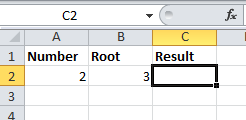Click the cell C2 and go to Formulas (main menu) –> Math & Trig and select POWER from the list.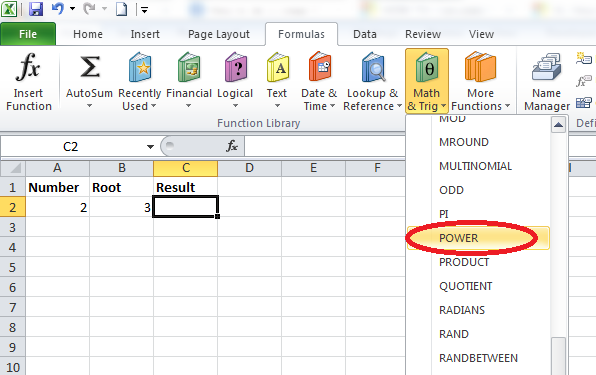You will get a window like this: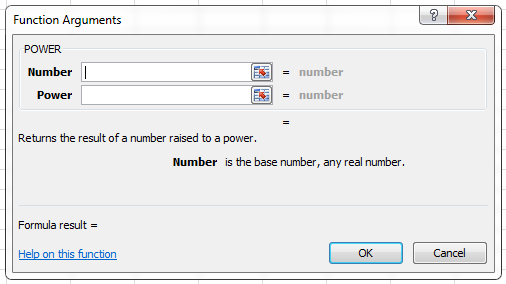Click inside the textbox next to Number and enter A2. Click the textbox next to Power and enter 1/B2. Now your screen will look like this: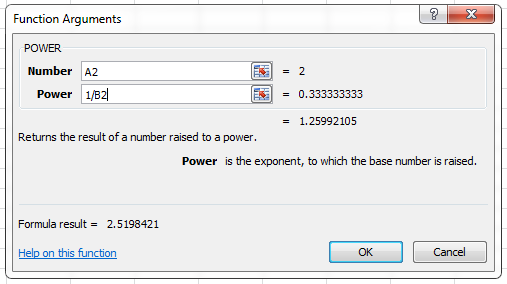Click OK and your screen will look like this.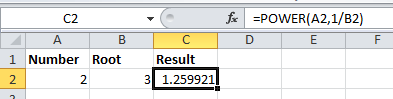=POWER(A2,1/B2)

To double check your result, copy the formula in C1 to C2 and enter 256 and 4 in the cells A3 and B3. You will get the result 4, that is the 4th root of 256 is equal to 4.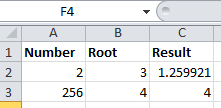You can use this generalized formula to calculate the nth root of any number. You just need to use the POWER function as POWER (x, 1/n) if you want to calculate the nth root of number x.

To calculate the nth root of a number in Excel manually, you can use the POWER function. The syntax for the POWER function is:

=POWER(number, power)

Where “number” is the number you want to find the nth root of, and “power” is the reciprocal of the nth root you want to find.

You can use any value for “number” and any value for “power” to calculate the nth root of a number in Excel.

Calculating the nth root of a number in Excel is a straightforward process that can be done using the POWER function. By providing the number and the reciprocal of the nth root as arguments, Excel can quickly return the desired result.

This feature can be particularly useful for mathematicians, statisticians, and anyone working with numerical data, as it provides an efficient way to calculate complex mathematical operations. Excel’s powerful computational abilities make it a valuable tool for anyone who needs to perform mathematical calculations on a regular basis.

## Calculate nth root of a number using a caret

An alternative method to calculate the Nth root of a number in Excel is to use the caret (^) operator along with the reciprocal of the Nth root. The syntax for this method is:

=number^(1/N)

Where “number” is the number you want to find the Nth root of, and “N” is the number of the root you want to find.

For example, to find the fourth root of 16 using this method, you would use the following formula:

=16^(1/4)

This returns the value 2, since the fourth root of 16 is 2.

Similarly, to find the cube root of 27 using this method, you would use the following formula:

=27^(1/3)

This returns the value 3, since the cube root of 27 is 3.

Both methods – using the POWER function and using the caret operator – can be used to calculate the Nth root of a number in Excel. Choosing one method over the other is a matter of personal preference.

## Calculate cube root of a number

To calculate the cube root of a number in Excel, you can use the POWER function with a fractional exponent of 1/3. The syntax for the POWER function is:

=POWER(number,1/3)

Where “number” is the number you want to find the cube root of.

For example, if you want to find the cube root of 64, you would use the following formula:

=POWER(64,1/3)

This returns the value 4, since the cube root of 64 is 4.

Similarly, if you want to find the cube root of a cell reference, you would use the following formula:

=POWER(A1,1/3)

This assumes that the number you want to find the cube root of is located in cell A1.

## How to calculate Square Root?

We are going to calculate the square root of a specific number. It is better to make sure that the number can be calculated easily. Put the number, which you would like to calculate its square root.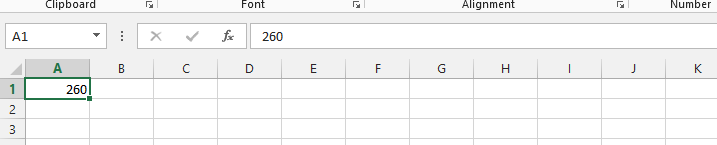To calculate the square root of a number in Excel, you can use the SQRT function. The syntax for the SQRT function is:

=SQRT(number)

Where “number” is the number you want to find the square root of. You can use any value for “number” or any cell reference that contains a number to calculate the square root in Excel.

Click on the column beside it, and type in =SQRT(column with the number that you would like to know the square root of, for example A1.)

Formula in the picture below is =SQRT(A1)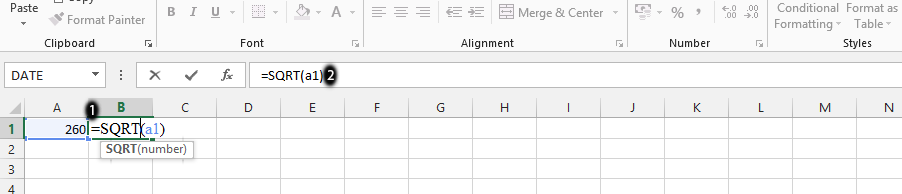Calculating the square root of a number in Excel is a simple process that can be done using the SQRT function. The SQRT function takes a single argument, which is the number you want to find the square root of. Excel then calculates the square root and returns the result.

This feature can be useful for a wide range of applications, from basic math operations to more complex data analysis. By using Excel’s built-in functions, users can save time and effort while performing mathematical calculations.